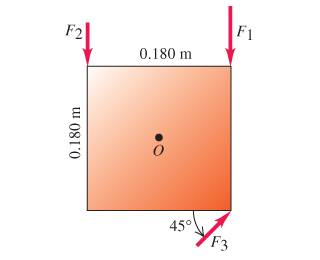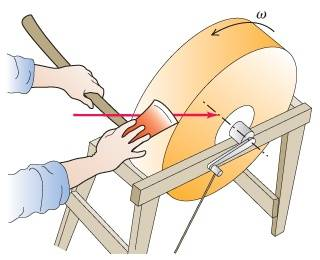# Moment of inertia (more) (1 Viewer)

### Users Who Are Viewing This Thread (Users: 0, Guests: 1)

#### cukitas2001

Ok in addition to my other post for help i have some other problems puzzling me.

1)A wagon wheel is constructed as shown in the figure. The radius of the wheel is 0.300 m, and the rim has mass 1.49 kg . Each of the eight spokes, that lie along a diameter and are 0.300 m long, has mass 0.220 kg.

What is the moment of inertia of the wheel about an axis through its center and perpendicular to the plane of the wheel?

Ok i know that the moment of inertia of just the outer rim is MR^2 but how do i take into account the Moment of inertia of the eight spokes?
I tried treating the spokes as if they were rods and using ML^2/12 and multiplying by 8 for the eight spokes but my answer came out wrong? so how is it supposed to be?

2) A uniform, solid disk with mass m and radius R is pivoted about a horizontal axis through its center. A small object of the same mass m is glued to the rim of the disk.

If the disk is released from rest with the small object at the end of a horizontal radius, find the angular speed when the small object is directly below the axis.

I have no idea where to begin on this any hints or tips?

3)A square metal plate 0.180 m on each side is pivoted about an axis through point O at its center and perpendicular to the plateCalculate the net torque about this axis due to the three forces shown in the figure if the magnitudes of the forces are F_1=18.0N, F_2=24.0N, and F_3=14.0N. The plate and all forces are in the plane of the page. Take positive torques to be counterclockwise.

Ok so i know that the effective lever arm r is perpendicular to O so for torque_1 is did T_1=18.0N*.09m=2.34 N*m and for T_2 similarly i did T_2=24N*.09m=1.62N*m but for T3 im stuck.... after i found all the individual torquest are they simply added together for net torque or must i multiply by and angle between them all?

4) A grindstone in the shape of a solid disk with diameter 0.460m and a mass of = 50.0kg is rotating at omega = 840 rev/min . You press an axe against the rim with a normal force of F = 220N, and the grindstone comes to rest in 7.00s .Ok i thought this one was going to be easy but i was wrong. I tried using W_other=-K1 since my other works canceled out. i converted omega into rad/s and for K1 i got -5116J. W_other should be the force times the distance it was done at correct? what i did was soemthing like d=r*t but i did d=omega*t and also tried using the tangent v=omega*r to find the distance that the friciton force acts to get its work.

Anyone see where im goin wrong or have a better method on this one?

#### cukitas2001

ok i figured out 1,3, and 4...any word on 2?

V

edit: late post

#### siddharth

##### What's this?
Homework Helper
Gold Member
2 looks like straight forward conservation of energy. What have you tried with 2?

#### cukitas2001

Conservation of energy makes sense but how can it be applied to 2?

#### Hootenanny

Staff Emeritus
Gold Member
cukitas2001 said:
Conservation of energy makes sense but how can it be applied to 2?
$\Delta$ Potential Energy = $\Delta$ Rotational Kinetic Energy

#### cukitas2001

ok i know that kinetic rotation energy is defined as: (1/2)I*omega^2 but whats potential in rotational motion? Please dont tell me its mgh or my head will explode#### Hootenanny

Staff Emeritus
Gold Member
cukitas2001 said:
ok i know that kinetic rotation energy is defined as: (1/2)I*omega^2 but whats potential in rotational motion? Please dont tell me its mgh or my head will explodeYes, it is indeed $mg\Delta h$, but in this case $\Delta h = R \Rightarrow E_{p} =mgR$. Don't let you head explode, brain is hell to get off a computer moniter!#### cukitas2001

This moment of inertia topic has been the hardest for me to get so far....i want to go back to collisions :!!) lol

Ok for my angular speed omega i got sqrt((4*g)/R) but it says im off by a multiplicative factor. Is there a way to take into account the part that says "when the small object is directly below the axis"? Is that where im going wrong?

#### Hootenanny

Staff Emeritus
Gold Member
cukitas2001 said:
This moment of inertia topic has been the hardest for me to get so far....i want to go back to collisions :!!) lol
No you don't rotational dynamics is awesome. :tongue2:
cukitas2001 said:
Ok for my angular speed omega i got sqrt((4*g)/R) but it says im off by a multiplicative factor. Is there a way to take into account the part that says "when the small object is directly below the axis"? Is that where im going wrong?
Don't forget that the small object will also have rotational kinetic energy (you can treat it as a point mass).

#### cukitas2001

What does it mean when you treat something as a point mass?

#### Hootenanny

Staff Emeritus
Gold Member
cukitas2001 said:
What does it mean when you treat something as a point mass?
A point mass is an object that has a mass but no volume. The moment of inertia of a point mass is therefore just I = mr2, where r is the distance from the axis of rotation.

#### cukitas2001

cool i have to remember that point mass rule

I have one last problem thats bothering me and if you could help i'd appreciate it astronomically:

A textbook of mass 1.99kg rests on a frictionless, horizontal surface. A cord attached to the book passes over a pulley whose diameter is 0.130m, to a hanging book with mass 2.90kg. The system is released from rest, and the books are observed to move a distance 1.16m over a time interval of 0.850s.

Part A) What is the tension in the part of the cord attached to the textbook?
Part B) What is the tension in the part of the cord attached to the book?

Okay so the tensions of the two should be the same since they are the same cord right? so what i tried doing was how it was done using Newtons second law. I drew freebody diagrams of the two and had two equations for both of the bodies. I calculated acceleration to be
a=5.81m/s^2 and pluging this back into the tension expressions i had form the free body diagrams i calculated tension to be 11.56N but it came back wrong? is this not the way to approach this problem?

#### Hootenanny

Staff Emeritus
Gold Member
cool i have to remember that point mass rule
That's just the standard form of the moment of inertia - $I = \sum mr^2$

However, how did you obtain your answer of a=5.81m/s2? I have achieved a different accleration.

Last edited:

#### cukitas2001

for the hanging mass i had an expression: 28.42N-T=2.90*a and then for the sliding body i had T=1.99*a adding both expressions and cancelling T i got 28.42N=4.89*a solving for a i get 5.81m/s^2

#### Hootenanny

Staff Emeritus
Gold Member
Sorry, just to correct myself from the previous post, the tension is not uniform. I forgot to take into account the moment of inertia of the pulley. Becuase the blocks are accelerating, the pulley must have angular accleration, therefore the two tensions must be different, therefore there is a different tension force acting on each block. I suggest setting up three different equations, one for each block and one for the pulley.

Last edited:

#### Hootenanny

Staff Emeritus
Gold Member
You seem to have gone quiet, so I'll start for you. Let T1 be the horizontal tension; T2 be the vertical tension. So;

Smaller Block

$$T_{1} = 1.99a$$

Larger Block

$$28.499 - T_{2}=2.9a$$

Pulley

$$\sum \tau = I\alpha = (T_{2} - T_{1})R$$

For the moment of inertia of the pulley you should use a disc.

Can you go from here?

#### cukitas2001

sorry i didnt even notice we were on page two....could you explain the expression you have for the pulley?

#### cukitas2001

the pulley equation is throwing me off some because from what i see there are two unknowns the mass of the pulley and alpha. Can i express alpha in terms of linear kinematics?

#### Hootenanny

Staff Emeritus
Gold Member
cukitas2001 said:
sorry i didnt even notice we were on page two....could you explain the expression you have for the pulley?
Certainly, it is know as Newton's second law of rotation;

$$\sum \tau = I\alpha$$

In words, the sum of the torques is equal to the product of the moment of inertia and and angular acceleration. Angular acceleration is given by the formula;

$$\alpha = \frac{a}{R}$$

Where a is linear acceleration and R is radius. Therefore, we can rewrite the original expression;

$$\sum \tau = I\cdot \frac{a}{R}$$

Now, torque($\tau$) (as you probably know) is given by the product of force and distance from the axis of rotation (R). The forces in this case are the tensions thus;

$$\sum \tau = T_{2}R - T_{1}R = I\cdot\frac{a}{R}$$

Factoring out the R we have;

$$(T_{2} - T_{1})R = I\cdot\frac{a}{R}$$

This is equivalent to the expression I gave originally, but in a more useful form. Do you follow?

### The Physics Forums Way

We Value Quality
• Topics based on mainstream science
• Proper English grammar and spelling
We Value Civility
• Positive and compassionate attitudes
• Patience while debating
We Value Productivity
• Disciplined to remain on-topic
• Recognition of own weaknesses
• Solo and co-op problem solving